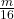## What is the equation for -2 = m divided by 16

Question

What is the equation for -2 = m divided by 16

in progress 0
5 months 2021-08-13T17:31:44+00:00 1 Answers 2 views 0

-2 =or= -2